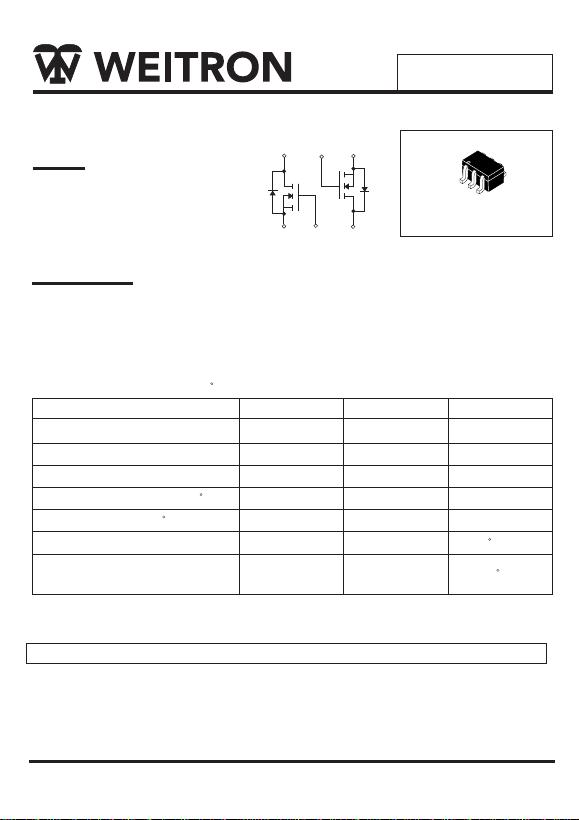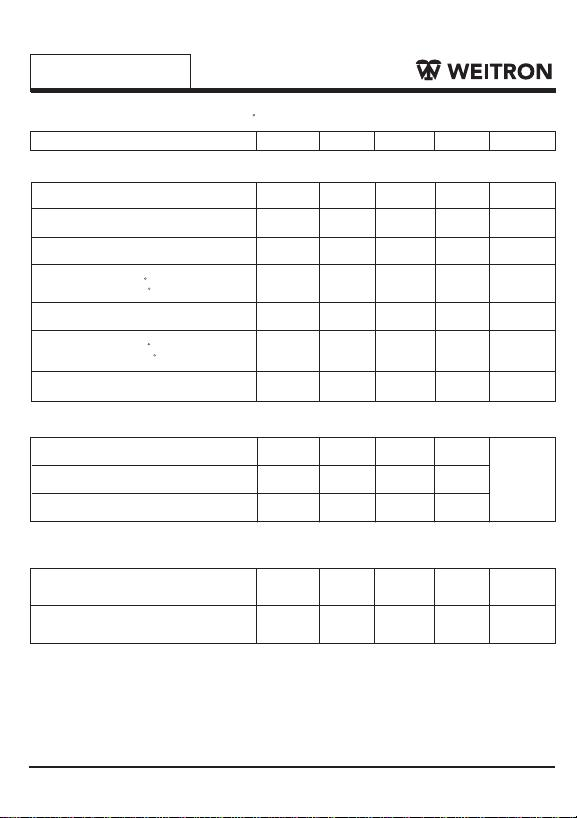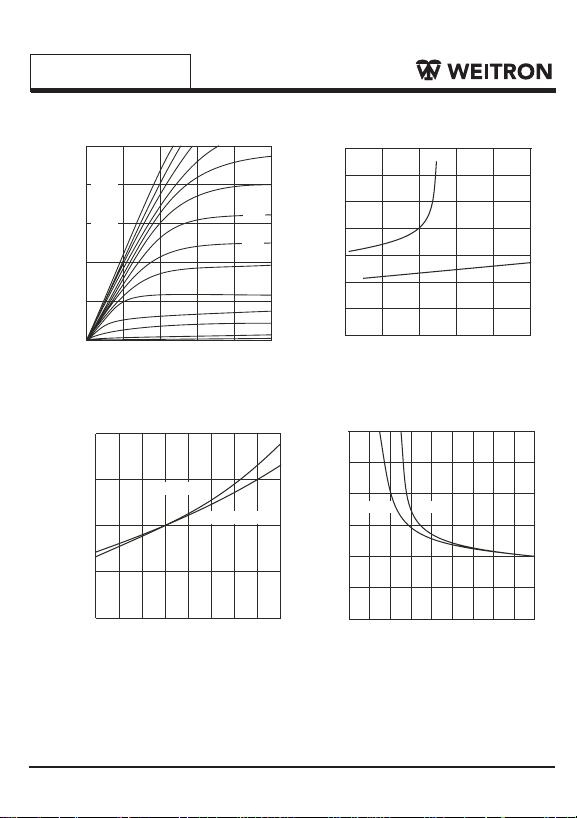WEITRON
http://www.weitron.com.tw
2N7002DW
Rating Symbol Value Unit
VDS
VGS
ID
PD
R JA
60
±20
115
200
625
Operating Junction and Storage
Temperature Range TJ, Tstg -55 to 150 C
V
V
V
mA
mW
C/W
Drain-Source Voltage
Gate-Source Voltage
Continuous Drain Current (TA=25 C)
Power Dissipation (TA=25 C)
Maximax Junction-to-Ambient
Maximum Ratings (TA=25 C Unless Otherwise Specified)
Device Marking
2N7002DW=
Note 1:
Pulse Width Limited by Maximum Junction Temperature
Dual N-Channel MOSFET
Features:
*Low On-Resistance : 7.5
*Low Input Capacitance: 22PF
*Low Out put Capacitance : 11PF
*Low Threshole :1 .5V(TYE)
*Fast Switching Speed : 11ns
Mechanical Data:
*Case: SOT-363, Molded Plastic
*Case Material-UL Flammability Rating 94V-0
*Terminals: Solderable per MIL-STD-202, Method 208
*Weight: 0.006 grams(approx.)
θ
Drain-Gate Voltage R <1.0M
GS _VDGR 60
SOT-363(SC-88)
123
654
45 6
1
2
3WEITRON
http://www.weitron.com.tw
2N7002DW
Input Capacitance
VDS=25V, VGS=0V, f=1MHZ
Output Capacitance
VDS=25V, VGS=0V, f=1MHZ
Reverse Transfer Capacitance
VDS=25V, VGS=0V, f=1MHZ
Drain-Source Breakdown Voltage
VGS=0V, ID=10 uA
Gate-Threshold Voltage
VDS=V , I =-250uA
Gate-body Leakage
VGS= 20V, VDS=0V
On-State Drain Current
VGS=10V, VDS=7.5V
Drain-Source On-Resistance
VGS=5V, ID=0.05A @
VGS=10V, ID=0.5A @
Forward Transconductance
VDS=10V, ID=0.2A
Static
Dynamic
Switching
Characteristic Symbol Unit
Min Typ Max
V(BR)DSS
VGS (th)
ID (on)
RDS (on)
IGSS
IDSS
gfs
Ciss
Coss
td(on)
td(off)
60 70 V
1.0 2.0 V
-
1.5
-
-
-
-
-
1.0
500
-
10 nA
uA
A
mS
0.5 1.0
3.2
4.4 7.5
50
25
5.0
22
11
2.0
PF
7.0
11
nS
nS
20
20
Electrical Characteristics (TA=25 C Unless otherwise noted)
Zero Gate Voltage Drain Current
-
-
-
80 --
-
-
-
-
-
Turn-On Time
VDD=30V, RL=150 ,ID=0.2A
VGEN=10V, RGEN=25
Turn-Off Time
VDD=30V, RL=150 , ID=0.2A
VGEN=10V, RGEN=25
GS D
_
++
_
Tc=25 C
Tc=125 C
Tj=25 C
Tj =125 C
VDS=60V, VGS=0V @
VDS=60V, VGS=0V @
13.5WEITRON
http://www.weitron.com.tw
2N7002DW
0
0.2
0.4
0.6
0.8
1
.
0
01 2 345
V = 10V
GS
9.0V
8.0V
7.0V
6.5V
6.0V
5.0V
4.5V
4.0V
3.5V
3.0V
2.5V
2.0/1.0V
5.5V
5.0V
V,DRAIN-SOURCE VOL TAGE (V)
DS
I,DRAIN-SOURCE CURRENT (A)
D
0
1
2
3
4
5
00.2
R,N
O
RMALIZED
DS(ON)
DRAIN-SOURCE ON-RESIST ANCE
I , DRAIN CURRENT (A)
D
V = 5.0V
GS
T=25°C
j
V = 10V
GS
6
7
0.4 0.6 0.8 1.0
0
0.5
1.0
1.5
2.0
-55 -30 -5 20 45 70 95 120 145
R,N
O
RMALIZED
DS(ON)
DRAIN-SOURCE ON-RESIST ANCE
T , JUNCTION TEMPERA TURE (°C)
j
VGS = 10V, I =
D0.5A
V = 5.0V , I = 0.05A
GS D
0
V , GATE TO SOURCE VOL TAGE (V)
GS
I
= 50mA
D
1
2
3
4
5
6
0 2 4 6 8 1012141618
R,NRMALIZED
DS(ON)
DRAIN-SOURCE ON-RESIST ANCE
I
= 500mA
D
FIG.1 On-Region Characteristics FIG.2 On-Resistance vs Drain Current
FIG.3 On-Resistance vs Junction Temperature FIG.4 On-Resistance vs. Gate-Source Voltage